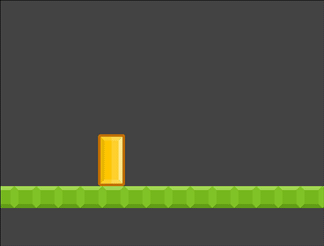# Kinematic Friction

## Problem

You want to add friction and acceleration to your kinematic character, giving it a smoother feel.

## Solution

For most games, we’re not necessarily interested in a perfect physics simulation. We want action, responsiveness, and arcade feel. This is why you choose a kinematic body over a rigid one: so that you can control its behavior directly. However, some amount of physics is good - it means an object doesn’t instantly change direction or come to a stop.

Below is the code for a no-frills kinematic platformer character:

``````extends KinematicBody2D

var speed = 1200
var jump_speed = -1800
var gravity = 4000

var velocity = Vector2.ZERO

func get_input():
velocity.x = 0
if Input.is_action_pressed("ui_right"):
velocity.x += speed
if Input.is_action_pressed("ui_left"):
velocity.x -= speed

func _physics_process(delta):
get_input()
velocity.y += gravity * delta
velocity = move_and_slide(velocity, Vector2.UP)
if Input.is_action_just_pressed("ui_select"):
if is_on_floor():
velocity.y = jump_speed
``````

If you run this code, you’ll see that the character’s x velocity changes instantaneously. To fix this, we’ll use `lerp()` to gradually increase/decrease the velocity.

#### Using `lerp`

``````lerp(start_value, end_value, amount)
``````

`lerp()`, aka linear interpolate, finds a “blended” value between two given numbers. See Interpolation for details.

In the code below, `friction` represents how quickly the character comes to a stop, while `acceleration` determines how quickly it gets up to full speed. Both are values between `0.0` and `1.0`.

Replace the `get_input()` code with the following:

``````var friction = 0.1
var acceleration = 0.5

func get_input():
var input_dir = 0
if Input.is_action_pressed("ui_right"):
input_dir += 1
if Input.is_action_pressed("ui_left"):
input_dir -= 1
if dir != 0:
# accelerate when there's input
velocity.x = lerp(velocity.x, dir * speed, acceleration)
else:
# slow down when there's no input
velocity.x = lerp(velocity.x, 0, friction)
``````

### Explanation

We’re using `friction` and `acceleration` as the amount to blend. For acceleration, we want to find a value between the current speed and the maximum, `speed`. When decelerating, we’re ramping the current speed down to `0`.